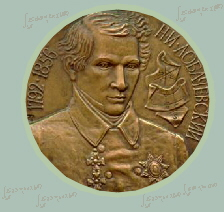LJM Editorial Board

Editor-in-Chief:   S. Novikov

 Editors: A. Aptekarev (Complex analysis & Function Theory) A. Elizarov (Complex analysis & Mathematical Physics) V. Vassiliev (Geometry & Topology) Coordinator: Yu. Hohlov (Complex Analysis) Secretary: M. Malakhaltsev (Geometry & Topology)

 Board members: F. Ablaev (Complexity Theory) F. Avhadiev (Complex Analysis); M. Arslanov (Logic & Complexity Theory) V. Voevodin (Computational Mathematics) V. Zharinov (Mathematical Physics) A. Kosterin (Mathematical Simulation) A. Lapin (Computational Mathematics) V. Lychagin (Geometry & Topology) D. Mushtari (Probability theory) A. Razborov (Logic & Complexity Theory) O. Tikhonov (Functional Analysis) E. Tyrtyshnikov (Computational Mathematics) A. Holevo (Theory of probability & Functional Analysis) B. Chetverushkin (Computational Mathematics) E. Chirka (Complex analysis & Geometry) Yi Zhang (Set theory & Algebra)

LJM homepage